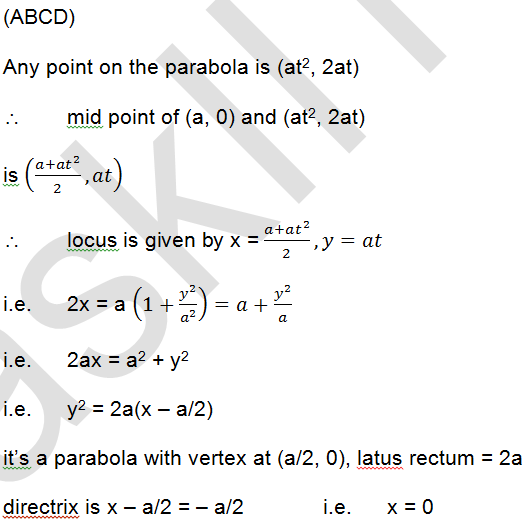# The locus of the mid point of the focal radii of a variable point moving on the parabola,            y2 = 4ax is a parabola whose            (a)       Latus rectum is half the latus rectum of the original parabola            (b)       Vertex is (a/2, 0)            (c)        Directrix is y-axis            (d)       Focus has the co-ordinates (a, 0)

9 years agoFocus is x – a/2 = a/2                      i.e.       x = a

i.e.       (a, 0)